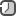### Types of machine learning algorithms

Regardless of whether the learner is a human or machine, the basic learning process is similar. It can be divided into four interrelated components:

• Data storage utilizes observation, memory, and recall to provide a factual basis for further reasoning.
• Abstraction involves the translation of stored data into broader representations and concepts.
• Generalization uses abstracted data to create knowledge and inferences that drive action in new contexts.
• Evaluation provides a feedback mechanism to measure the utility of learned knowledge and inform potential improvements.

Machine learning algorithms are divided into categories according to their purpose.

Main categories are

• Supervised learning (predictive model, "labeled" data)
• classification (Logistic Regression, Decision Tree, KNN, Random Forest, SVM, Naive Bayes, etc)
• numeric prediction (Linear Regression, KNN, Gradient Boosting & AdaBoost, etc)
• Unsupervised learning (descriptive model, "unlabeled" data)
• clustering (K-Means)
• pattern discovery
• Semi-supervised learning (mixture of "labeled" and "unlabeled" data).
• Reinforcement learning. Using this algorithm, the machine is trained to make specific decisions. It works this way: the machine is exposed to an environment where it trains itself continually using trial and error. This machine learns from past experience and tries to capture the best possible knowledge to make accurate business decisions. Example of Reinforcement Learning: Markov Decision Process.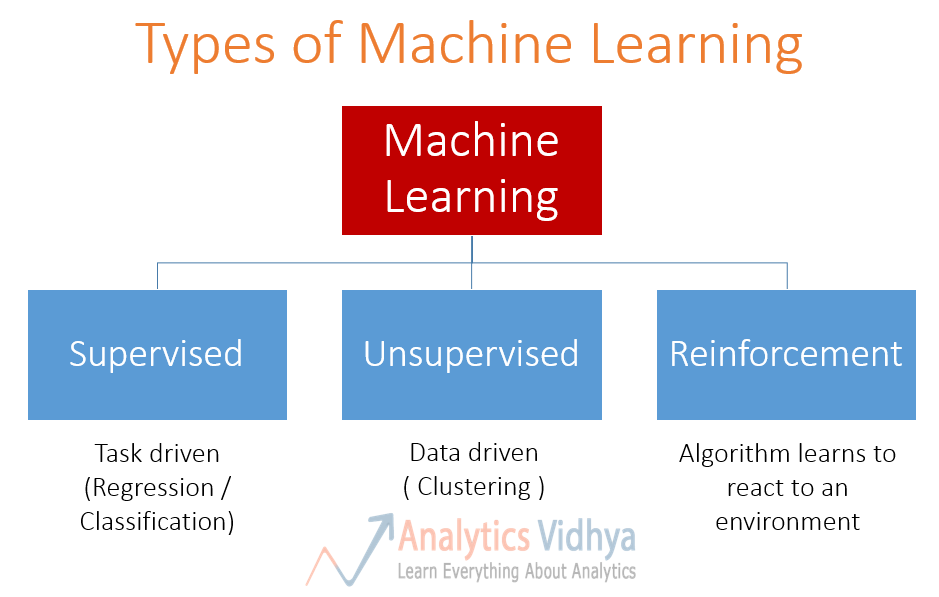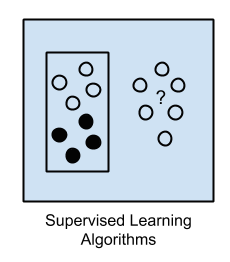There are lots of overlaps in which ML algorithms are applied to a particular problem. As a result, for the same problem, there could be many different ML models possible. So, coming out with the best ML model is an art that requires a lot of patience and trial and error. Following figure provides a brief of all these learning types with sample use cases.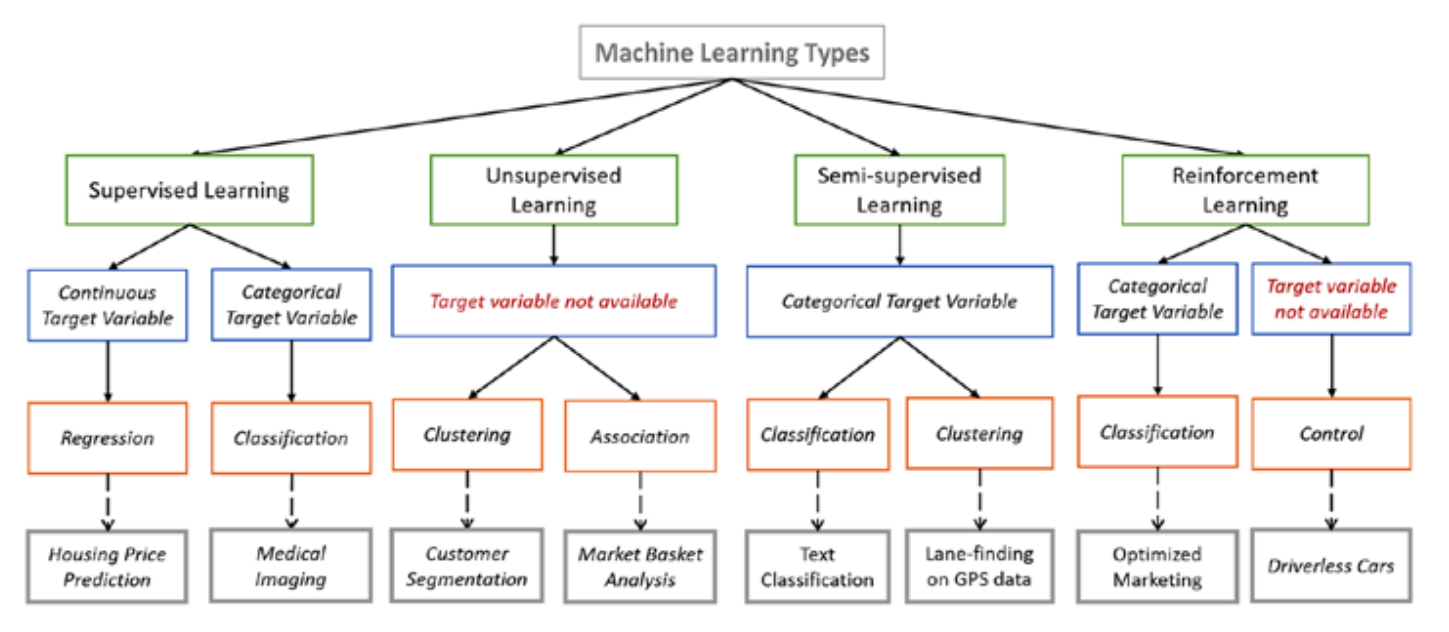The supervised learning algorithms are a subset of the family of machine learning algorithms which are mainly used in predictive modeling. A predictive model is basically a model constructed from a machine learning algorithm and features or attributes from training data such that we can predict a value using the other values obtained from the input data. Supervised learning algorithms try to model relationships and dependencies between the target prediction output and the input features such that we can predict the output values for new data based on those relationships which it learned from the previous data sets. The main types of supervised learning algorithms include:

• Classification algorithms: These algorithms build predictive models from training data which have features and class labels. These predictive models in-turn use the features learnt from training data on new, previously unseen data to predict their class labels. The output classes are discrete. Types of classification algorithms include decision trees, random forests, support vector machines, and many more.
• Regression algorithms: These algorithms are used to predict output values based on some input features obtained from the data. To do this, the algorithm builds a model based on features and output values of the training data and this model is used to predict values for new data. The output values in this case are continuous and not discrete. Types of regression algorithms include linear regression, multivariate regression, regression trees, and lasso regression, among many others.

Some application of supervised learning are speech recognition, credit scoring, medical imaging, and search engines.

The unsupervised learning algorithms are the family of machine learning algorithms which are mainly used in pattern detection and descriptive modeling. However, there are no output categories or labels here based on which the algorithm can try to model relationships. These algorithms try to use techniques on the input data to mine for rules, detect patterns, and summarize and group the data points which help in deriving meaningful insights and describe the data better to the users. The main types of unsupervised learning algorithms include:

• Clustering algorithms: The main objective of these algorithms is to cluster or group input data points into different classes or categories using just the features derived from the input data alone and no other external information. Unlike classification, the output labels are not known beforehand in clustering. There are different approaches to build clustering models, such as by using means, medoids, hierarchies, and many more. Some popular clustering algorithms include k-means, k-medoids, and hierarchical clustering.
• Association rule learning algorithms: These algorithms are used to mine and extract rules and patterns from data sets. These rules explain relationships between different variables and attributes, and also depict frequent item sets and patterns which occur in the data. These rules in turn help discover useful insights for any business or organization from their huge data repositories. Popular algorithms include Apriori and FP Growth.

Some applications of unsupervised learning are customer segmentation in marketing, social network analysis, image segmentation, climatology, and many more.

Semi-Supervised Learning. In the previous two types, either there are no labels for all the observation in the dataset or labels are present for all the observations. Semi-supervised learning falls in between these two. In many practical situations, the cost to label is quite high, since it requires skilled human experts to do that. So, in the absence of labels in the majority of the observations but present in few, semi-supervised algorithms are the best candidates for the model building. These methods exploit the idea that even though the group memberships of the unlabeled data are unknown, this data carries important information about the group parameters.

The reinforcement learning method aims at using observations gathered from the interaction with the environment to take actions that would maximize the reward or minimize the risk. Reinforcement learning algorithm (called the agent) continuously learns from the environment in an iterative fashion. In the process, the agent learns from its experiences of the environment until it explores the full range of possible states.

In order to produce intelligent programs (also called agents), reinforcement learning goes through the following steps:

1. Input state is observed by the agent.
2. Decision making function is used to make the agent perform an action.
3. After the action is performed, the agent receives reward or reinforcement from the environment.
4. The state-action pair information about the reward is stored.

Some applications of the reinforcement learning algorithms are computer played board games (Chess, Go), robotic hands, and self-driving cars.

Predictive model

A predictive model is used for tasks that involve the prediction of one value using other values in the dataset. The learning algorithm attempts to discover and model the relationship between the target feature (the feature being predicted) and the other features. Despite the common use of the word "prediction" to imply forecasting, predictive models need not necessarily foresee events in the future. For instance, a predictive model could be used to predict past events, such as the date of a baby's conception using the mother's present-day hormone levels. Predictive models can also be used in real time to control traffic lights during rush hours.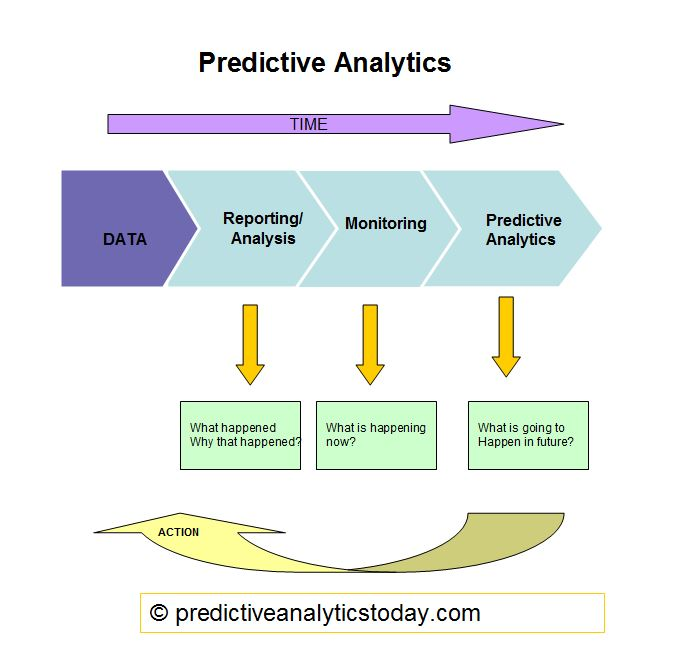Because predictive models are given clear instruction on what they need to learn and how they are intended to learn it, the process of training a predictive model is known as supervised learning. The supervision does not refer to human involvement, but rather to the fact that the target values provide a way for the learner to know how well it has learned the desired task. Stated more formally, given a set of data, a supervised learning algorithm attempts to optimize a function (the model) to find the combination of feature values that result in the target output.So, supervised learning consist of a target / outcome variable (or dependent variable) which is to be predicted from a given set of predictors (independent variables). Using these set of variables, we generate a function that map inputs to desired outputs. The training process continues until the model achieves a desired level of accuracy on the training data. Examples of Supervised Learning: Regression, Decision Tree, Random Forest, KNN, Logistic Regression etc.

The often used supervised machine learning task of predicting which category an example belongs to is known as classification. It is easy to think of potential uses for a classifier. For instance, you could predict whether:

• An e-mail message is spam
• A person has cancer
• A football team will win or lose
• An applicant will default on a loan

In classification, the target feature to be predicted is a categorical feature known as the class, and is divided into categories called levels. A class can have two or more levels, and the levels may or may not be ordinal. Because classification is so widely used in machine learning, there are many types of classification algorithms, with strengths and weaknesses suited for different types of input data.Supervised learners can also be used to predict numeric data such as income, laboratory values, test scores, or counts of items. To predict such numeric values, a common form of numeric prediction fits linear regression models to the input data. Although regression models are not the only type of numeric models, they are, by far, the most widely used. Regression methods are widely used for forecasting, as they quantify in exact terms the association between inputs and the target, including both, the magnitude and uncertainty of the relationship.

Descriptive model

A descriptive model is used for tasks that would benefit from the insight gained from summarizing data in new and interesting ways. As opposed to predictive models that predict a target of interest, in a descriptive model, no single feature is more important than any other. In fact, because there is no target to learn, the process of training a descriptive model is called unsupervised learning. Although it can be more difficult to think of applications for descriptive models, what good is a learner that isn't learning anything in particular - they are used quite regularly for data mining.

So, in unsupervised learning algorithm, we do not have any target or outcome variable to predict / estimate. It is used for clustering population in different groups, which is widely used for segmenting customers in different groups for specific intervention. Examples of Unsupervised Learning: Apriori algorithm, K-means.

For example, the descriptive modeling task called pattern discovery is used to identify useful associations within data. Pattern discovery is often used for market basket analysis on retailers' transactional purchase data. Here, the goal is to identify items that are frequently purchased together, such that the learned information can be used to refine marketing tactics. For instance, if a retailer learns that swimming trunks are commonly purchased at the same time as sunglasses, the retailer might reposition the items more closely in the store or run a promotion to "up-sell" customers on associated items.

The descriptive modeling task of dividing a dataset into homogeneous groups is called clustering. This is sometimes used for segmentation analysis that identifies groups of individuals with similar behavior or demographic information, so that advertising campaigns could be tailored for particular audiences. Although the machine is capable of identifying the clusters, human intervention is required to interpret them. For example, given five different clusters of shoppers at a grocery store, the marketing team will need to understand the differences among the groups in order to create a promotion that best suits each group.Lastly, a class of machine learning algorithms known as meta-learners is not tied to a specific learning task, but is rather focused on learning how to learn more effectively. A meta-learning algorithm uses the result of some learnings to inform additional learning. This can be beneficial for very challenging problems or when a predictive algorithm's performance needs to be as accurate as possible.

The following table lists only a fraction of the entire set of machine learning algorithms.

Model Learning task
Supervised Learning Algorithms
Nearest Neighbor Classification
Naive Bayes Classification
Decision Trees Classification
Classification Rule Learners Classification
Linear Regression Numeric prediction
Model Trees Numeric prediction
Regression Trees
Neural Networks Dual use
Support Vector Machines Dual use
Unsupervised Learning Algorithms
Association Rules Pattern detection
k-means clustering Clustering
Meta-Learning Algorithms
Bagging Dual use
Boosting Dual use
Random Forests Dual use

To begin applying machine learning to a real-world project, you will need to determine which of the four learning tasks your project represents: classification, numeric prediction, pattern detection, or clustering. The task will drive the choice of algorithm. For instance, if you are undertaking pattern detection, you are likely to employ association rules. Similarly, a clustering problem will likely utilize the k-means algorithm, and numeric prediction will utilize regression analysis or regression trees.

Torsten Hothorn maintains an exhaustive list of packages available in R for implementing machine learning algorithms.

Model evaluation

Whenever we are building a model, it needs to be tested and evaluated to ensure that it will not only work on trained data, but also on unseen data and can generate results with accuracy. A model should not generate a random result though some noise is permitted. If the model is not evaluated properly then the chances are that the result produced with unseen data is not accurate. Furthermore, model evaluation can help select the optimum model, which is more robust and can accurately predict responses for future subjects.

There are various ways by which a model can be evaluated:

• Split test. In a split test, the dataset is divided into two parts, one is the training set and the other is test dataset. Once data is split the algorithm will use the training set and a model is created. The accuracy of a model is tested using the test dataset. The ratio of dividing the dataset in training and test can be decided on basis of the size of the dataset. It is fast and great when the dataset is of large size or the dataset is expensive. It can produce different result on how the dataset is divided into the training and test dataset. If the date set is divided in 80% as a training set and 20% as a test set, 60% as a training set and 40%, both will generate different results. We can go for multiple split tests, where the dataset is divided in different ratios and the result is found and compared for accuracy.
• Cross validation. In cross validation, the dataset is divided in number of parts, for example, dividing the dataset in 10 parts. An algorithm is run on 9 subsets and holds one back for test. This process is repeated 10 times. Based on different results generated on each run, the accuracy is found. It is known as k-fold cross validation is where k is the number in which a dataset is divided. Selecting the k is very crucial here, which is dependent on the size of dataset.
• Bootstrap. We start with some random samples from the dataset, and an algorithm is run on dataset. This process is repeated for n times until we have all covered the full dataset. In aggregate, the result provided in all repetition shows the model performance.
• Leave One Out Cross Validation. As the name suggests, only one data point from the dataset is left out, an algorithm is run on the rest of the dataset and it is repeated for each point. As all points from the dataset are covered it is less biased, but it requires higher execution time if the dataset is large.

Model evaluation is a key step in any machine learning process. It is different for supervised and unsupervised models. In supervised models, predictions play a major role; whereas in unsupervised models, homogeneity within clusters and heterogeneity across clusters play a major role.

Some widely used model evaluation parameters for regression models (including cross validation) are as follows:

• Coefficient of determination
• Root mean squared error
• Mean absolute error
• Akaike or Bayesian information criterion

Some widely used model evaluation parameters for classification models (including cross validation) are as follows:

• Confusion matrix (accuracy, precision, recall, and F1-score)
• Gain or lift charts
• Area under ROC (receiver operating characteristic) curve
• Concordant and discordant ratio

Some of the widely used evaluation parameters of unsupervised models (clustering) are as follows:

• Contingency tables
• Sum of squared errors between clustering objects and cluster centers or centroids
• Silhouette value
• Rand index
• Matching index
• Pairwise and adjusted pairwise precision and recall (primarily used in NLP)

Bias and variance are two key error components of any supervised model; their trade-off plays a vital role in model tuning and selection. Bias is due to incorrect assumptions made by a predictive model while learning outcomes, whereas variance is due to model rigidity toward the training dataset. In other words, higher bias leads to underfitting and higher variance leads to overfitting of models.

In bias, the assumptions are on target functional forms. Hence, this is dominant in parametric models such as linear regression, logistic regression, and linear discriminant analysis as their outcomes are a functional form of input variables.

Variance, on the other hand, shows how susceptible models are to change in datasets. Generally, target functional forms control variance. Hence, this is dominant in non-parametric models such as decision trees, support vector machines, and K-nearest neighbors as their outcomes are not directly afunctional form of input variables. In other words, the hyperparameters of non-parametric models can lead to overfitting of predictive models.

Useful links